# LeetCode 专题暴击：BFS真的就是照猫画虎

### 前言

BFS 有一个很经典的模板讨论，在python代码中，我们可以简单的使用queue写出他的模板公式：

``````def bfs():
q = collections.deque()
Step 1: q 放入第一个元素
while q:
cur = q.popleft()
Step 2: 处理cur的所有邻居
Step 3: 判断范围和符合情况
q.append(邻居)``````

### 二叉树的BFS

#### 二叉树的BFS模板

``````def bfs(root):
if not root:
return
q = collections.deque()
q.append(root)
while q:
cur = q.popleft()
# do something with cur
if cur.left:
q.append(cur.left)
if cur.right:
q.append(cur.right)``````

#### 112. Path Sum

test cases: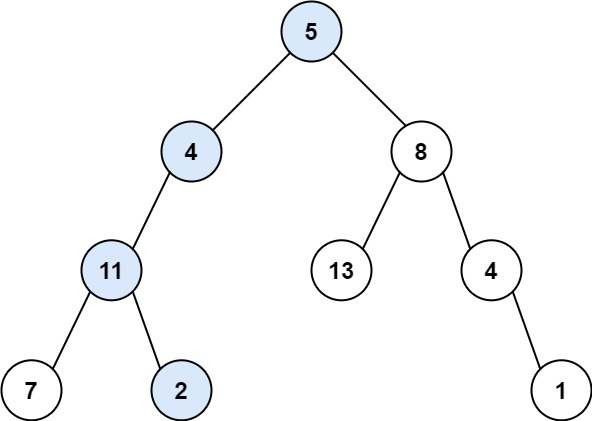``````Input: root = [5,4,8,11,null,13,4,7,2,null,null,null,1], targetSum = 22
Output: true
Explanation: The root-to-leaf path with the target sum is shown.``````

``````class Solution:
def hasPathSum(self, root: TreeNode, sum: int) -> bool:
if not root:
return False
q = collections.deque()
q.append(root)
while q:
cur = q.popleft()
if not cur.left and not cur.right and cur.val == sum:
return True
if cur.left:
cur.left.val += cur.val
q.append(cur.left)
if cur.right:
cur.right.val += cur.val
q.append(cur.right)
return False``````

• 时间复杂度：O(N)，其中 N 是树的节点数。对每个节点访问一次。
• 空间复杂度：O(N)，其中 N 是树的节点数。空间复杂度主要取决于队列的开销，队列中的元素个数不会超过树的节点数。

#### 111. Minimum Depth of Binary Tree

test cases: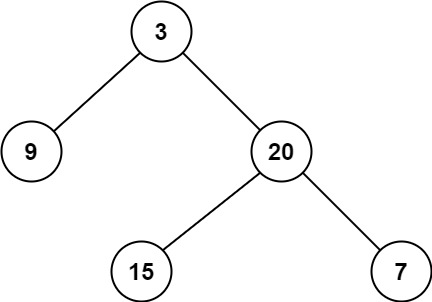``````Input: root = [3,9,20,null,null,15,7]
Output: 2``````

``````class Solution:
def minDepth(self, root: TreeNode) -> int:
if not root:
return 0
q = collections.deque()
q.append(root)
depth = 0
while q:
depth += 1
size = len(q)
for _ in range(size):
cur = q.popleft()
if not cur.left and not cur.right:
return depth
if cur.left:
q.append(cur.left)
if cur.right:
q.append(cur.right)
return depth``````

• 时间复杂度：O(N)，其中 N 是树的节点数。对每个节点访问一次。
• 空间复杂度：O(N)，其中 N 是树的节点数。空间复杂度主要取决于队列的开销，队列中的元素个数不会超过树的节点数。

#### 104. Maximum Depth of Binary Tree

test cases: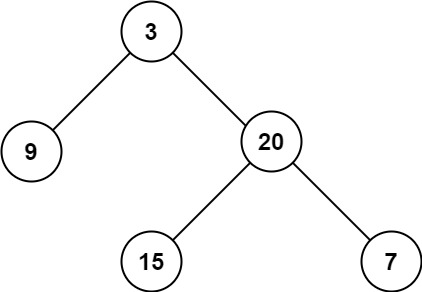``````Input: root = [3,9,20,null,null,15,7]
Output: 3``````

``````class Solution:
def maxDepth(self, root: TreeNode) -> int:
if not root:
return 0
q = collections.deque()
q.append(root)
depth = 0
while q:
depth += 1
size = len(q)
for _ in range(size):
cur = q.popleft()
if cur.left:
q.append(cur.left)
if cur.right:
q.append(cur.right)
return depth``````

• 时间复杂度：O(N)，其中 N 是树的节点数。对每个节点访问一次。
• 空间复杂度：O(N)，其中 N 是树的节点数。空间复杂度主要取决于队列的开销，队列中的元素个数不会超过树的节点数。

#### 129. Sum Root to Leaf Numbers

test cases:``````Input: root = [4,9,0,5,1]
Output: 1026
Explanation:
The root-to-leaf path 4->9->5 represents the number 495.
The root-to-leaf path 4->9->1 represents the number 491.
The root-to-leaf path 4->0 represents the number 40.
Therefore, sum = 495 + 491 + 40 = 1026.``````

``````class Solution:
def sumNumbers(self, root: TreeNode) -> int:
if not root:
return 0
q = collections.deque()
q.append(root)
res = 0
while q:
size = len(q)
for _ in range(size):
cur = q.popleft()
if not cur.left and not cur.right:
res += cur.val
if cur.left:
cur.left.val += cur.val * 10 # 这里是关键，每次都要乘以10
q.append(cur.left)
if cur.right:
cur.right.val += cur.val * 10 # 这里是关键，每次都要乘以10
q.append(cur.right)
return res``````

``````class Solution:
def sumNumbers(self, root: Optional[TreeNode]) -> int:
if not root:
return 0

q = collections.deque([(root, str(root.val))])

res = []
while q:
cur, path = q.popleft()
if not cur.left and not cur.right:
res.append(path)

elif not cur.left and cur.right:
q.append((cur.right, path + str(cur.right.val)))

elif cur.left and not cur.right:
q.append((cur.left, path + str(cur.left.val)))

else:
q.append((cur.right, path + str(cur.right.val)))
q.append((cur.left, path + str(cur.left.val)))

return sum([int(path) for path in res]) # 也可以不用sum，直接在上面的if not cur.left and not cur.right:里面直接res += int(path)``````

• 时间复杂度：O(N)，其中 N 是树的节点数。对每个节点访问一次。
• 空间复杂度：O(N)，其中 N 是树的节点数。空间复杂度主要取决于队列的开销，队列中的元素个数不会超过树的节点数。

#### 102. Binary Tree Level Order Traversal

``````class Solution:
def levelOrder(self, root: TreeNode) -> List[List[int]]:
if not root:
return []
q = collections.deque()
q.append(root) # Step 1: q 放入root元素
res = []
while q:
size = len(q) # 这里需要注意，我们需要先把当前层的size存下来，因为后面q的size会变化
level = []
for _ in range(size): # 使用for循环来遍历当前层的所有元素
cur = q.popleft()  # Step 2: 处理cur的所有邻居
level.append(cur.val)
if cur.left:
q.append(cur.left)
if cur.right:
q.append(cur.right)
res.append(level) # Step 3: 把当前层的结果放入res
return res``````

• 时间复杂度：O(N)，其中 N 是树的节点数。对每个节点访问一次。
• 空间复杂度：O(N)，其中 N 是树的节点数。空间复杂度主要取决于队列的开销，队列中的元素个数不会超过树的节点数。

#### 107. Binary Tree Level Order Traversal II

``````class Solution:
def levelOrderBottom(self, root: TreeNode) -> List[List[int]]:
if not root:
return []
q = collections.deque()
q.append(root) # Step 1: q 放入root元素
res = []
while q:
size = len(q) # 这里需要注意，我们需要先把当前层的size存下来，因为后面q的size会变化
level = []
for _ in range(size): # 使用for循环来遍历当前层的所有元素
cur = q.popleft()  # Step 2: 处理cur的所有邻居
level.append(cur.val)
if cur.left:
q.append(cur.left)
if cur.right:
q.append(cur.right)
res.append(level) # Step 3: 把当前层的结果放入res
return res[::-1]``````

• 时间复杂度：O(N)，其中 N 是树的节点数。对每个节点访问一次。
• 空间复杂度：O(N)，其中 N 是树的节点数。空间复杂度主要取决于队列的开销，队列中的元素个数不会超过树的节点数。

#### 103. Binary Tree Zigzag Level Order Traversal

test cases: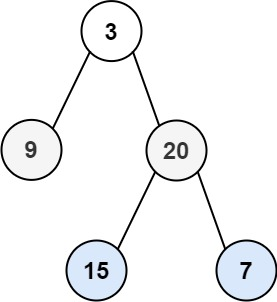``````Input: root = [3,9,20,null,null,15,7]
Output: [,[20,9],[15,7]]``````

``````class Solution:
def zigzagLevelOrder(self, root: TreeNode) -> List[List[int]]:
if not root:
return []
q = collections.deque()
q.append(root) # Step 1: q 放入root元素
res = []
while q:
size = len(q) # 这里需要注意，我们需要先把当前层的size存下来，因为后面q的size会变化
level = []
for _ in range(size): # 使用for循环来遍历当前层的所有元素
cur = q.popleft()  # Step 2: 处理cur的所有邻居
level.append(cur.val)
if cur.left:
q.append(cur.left)
if cur.right:
q.append(cur.right)
if len(res) % 2 == 1: # 如果是奇数层，我们就把当前层的结果翻转一下
level = level[::-1]
res.append(level)
return res``````

• 时间复杂度：O(N)，其中 N 是树的节点数。对每个节点访问一次。
• 空间复杂度：O(N)，其中 N 是树的节点数。空间复杂度主要取决于队列的开销，队列中的元素个数不会超过树的节点数。

#### 199. Binary Tree Right Side View

test cases: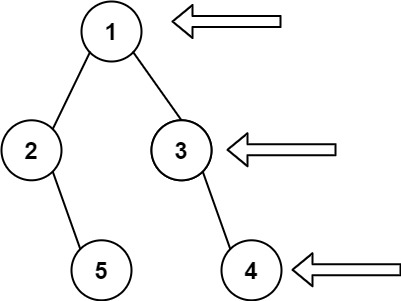``````Input: root = [1,2,3,null,5,null,4]
Output: [1,3,4]``````

``````class Solution:
def rightSideView(self, root: TreeNode) -> List[int]:
if not root:
return []
q = collections.deque()
q.append(root) # Step 1: q 放入root元素
res = []
while q:
size = len(q) # 这里需要注意，我们需要先把当前层的size存下来，因为后面q的size会变化
level = []
for _ in range(size): # 使用for循环来遍历当前层的所有元素
cur = q.popleft()  # Step 2: 处理cur的所有邻居
level.append(cur.val)
if cur.left:
q.append(cur.left)
if cur.right:
q.append(cur.right)
res.append(level[-1]) # 把当前层的最后一个元素加入到结果中
return res``````

• 时间复杂度：O(N)，其中 N 是树的节点数。对每个节点访问一次。
• 空间复杂度：O(N)，其中 N 是树的节点数。空间复杂度主要取决于队列的开销，队列中的元素个数不会超过树的节点数。

#### 116. Populating Next Right Pointers in Each Node

test cases: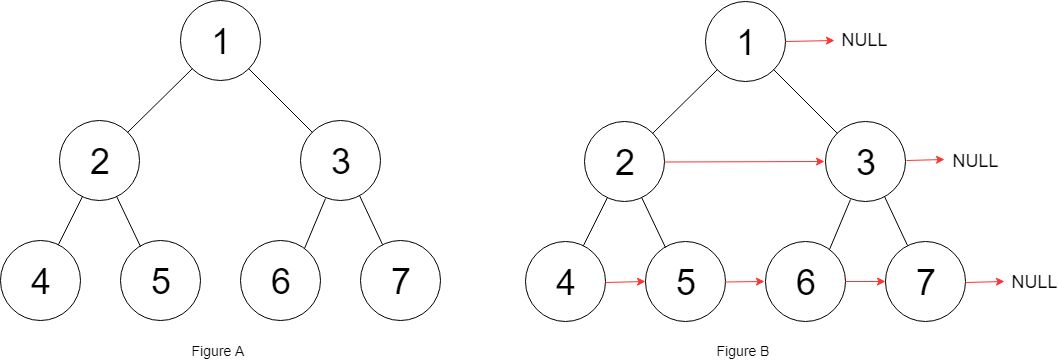``````Input: root = [1,2,3,4,5,6,7]
Output: [1,#,2,3,#,4,5,6,7,#]
Explanation: Given the above perfect binary tree (Figure A), your function should populate each next pointer to point to its next right node, just like in Figure B. The serialized output is in level order as connected by the next pointers, with '#' signifying the end of each level.``````

``````class Solution:
def connect(self, root: 'Node') -> 'Node':
if not root:
return root
q = collections.deque()
q.append(root) # Step 1: q 放入root元素
while q:
size = len(q) # 这里需要注意，我们需要先把当前层的size存下来，因为后面q的size会变化
for i in range(size): # 使用for循环来遍历当前层的所有元素
cur = q.popleft()  # Step 2: 处理cur的所有邻居
if i < size - 1: # 如果不是当前层的最后一个元素，那么就把cur的next指向q
cur.next = q
if cur.left:
q.append(cur.left)
if cur.right:
q.append(cur.right)
return root``````

• 时间复杂度：O(N)，其中 N 是树的节点数。对每个节点访问一次。
• 空间复杂度：O(N)，其中 N 是树的节点数。空间复杂度主要取决于队列的开销，队列中的元素个数不会超过树的节点数。

#### 117. Populating Next Right Pointers in Each Node II

test cases: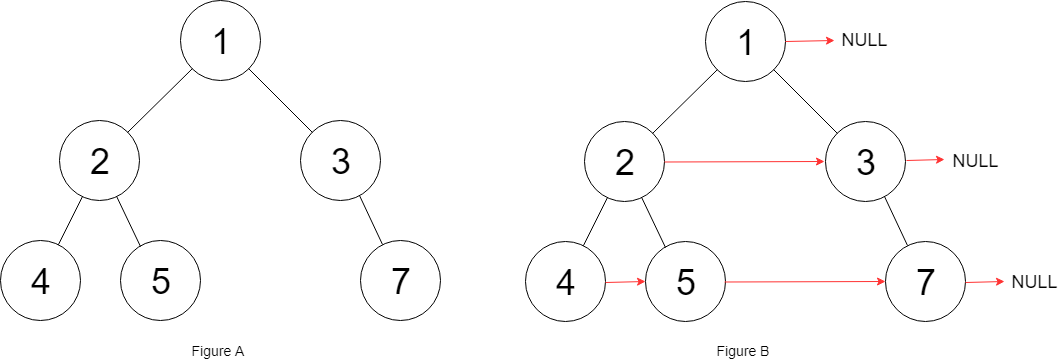``````Input: root = [1,2,3,4,5,null,7]
Output: [1,#,2,3,#,4,5,7,#]
Explanation: Given the above binary tree (Figure A), your function should populate each next pointer to point to its next right node, just like in Figure B. The serialized output is in level order as connected by the next pointers, with '#' signifying the end of each level.``````

``````class Solution:
def connect(self, root: 'Node') -> 'Node':
if not root:
return root
q = collections.deque()
q.append(root) # Step 1: q 放入root元素
while q:
size = len(q) # 这里需要注意，我们需要先把当前层的size存下来，因为后面q的size会变化
for i in range(size): # 使用for循环来遍历当前层的所有元素
cur = q.popleft()  # Step 2: 处理cur的所有邻居
if i < size - 1: # 如果不是当前层的最后一个元素，那么就把cur的next指向q
cur.next = q
if cur.left:
q.append(cur.left)
if cur.right:
q.append(cur.right)
return root``````

• 时间复杂度：O(N)，其中 N 是树的节点数。对每个节点访问一次。
• 空间复杂度：O(N)，其中 N 是树的节点数。空间复杂度主要取决于队列的开销，队列中的元素个数不会超过树的节点数。

### 矩阵的BFS

#### 200. Number of Islands

test cases:

``````Input: grid = [
["1","1","0","0","0"],
["1","1","0","0","0"],
["0","0","1","0","0"],
["0","0","0","1","1"]
]
Output: 3``````

``````class Solution:
def numIslands(self, grid: List[List[str]]) -> int:
if not grid:
return grid

row = len(grid)
col = len(grid)
count = 0
visited = [[0 for _ in range(col)] for _ in range(row)] # 用来标记是否访问过

def bfs(grid, i, j):
q = collections.deque([(i, j)])
visited[i][j] = 1

while q:
cur_x, cur_y = q.popleft()
for x, y in (cur_x + 1, cur_y), (cur_x - 1, cur_y), (cur_x, cur_y + 1), (cur_x, cur_y - 1): # 上下左右四个方向
if 0 <= x < row and 0 <= y < col and not visited[x][y] and grid[x][y] == "1": # 如果是陆地，那么就把它放入队列中
q.append((x, y))
visited[x][y] = 1

for i in range(row):
for j in range(col):
if not visited[i][j] and grid[i][j] == "1":
bfs(grid, i, j)
count += 1

return count``````

``````class Solution:
def numIslands(self, grid: List[List[str]]) -> int:
if not grid:
return grid

row = len(grid)
col = len(grid)
count = 0

def bfs(grid, i, j):
q = collections.deque([(i, j)])
grid[i][j] = "#" # 标记已经访问过的陆地

while q:
cur_x, cur_y = q.popleft()
for x, y in (cur_x + 1, cur_y), (cur_x - 1, cur_y), (cur_x, cur_y + 1), (cur_x, cur_y - 1): # 上下左右四个方向
if 0 <= x < row and 0 <= y < col and grid[x][y] == "1": # 如果是陆地，那么就把它放入队列中
q.append((x, y))
grid[x][y] = "#"

for i in range(row):
for j in range(col):
if grid[i][j] == "1":
bfs(grid, i, j)
count += 1

return count``````

• 时间复杂度：O(MN)，其中 M 和 N 分别为行数和列数。因为我们最多遍历一次矩阵中的全部元素。
• 空间复杂度：O(min(M,N))，在最坏的情况下（全部为陆地），队列的大小可以达到 min(M,N)。

#### 542. 01 Matrix

test cases:

``````Input:
[[0,0,0],
[0,1,0],
[1,1,1]]

Output:
[[0,0,0],
[0,1,0],
[1,2,1]]``````

``````class Solution:
def updateMatrix(self, mat: List[List[int]]) -> List[List[int]]:
q = deque()
visited = set()

for i in range(len(mat)):
for j in range(len(mat)):
if mat[i][j] == 0:
q.append((i, j)) # 把所有的海洋都当做是起始点

while q:
i, j = q.popleft()

for x, y in (i + 1, j), (i - 1, j), (i, j + 1), (i, j - 1):
if 0 <= x < len(mat) and 0 <= y < len(mat) and (x, y) not in visited:
mat[x][y] = mat[i][j] + 1
q.append((x, y))

return mat``````

• 时间复杂度：O(MN)，其中 M 和 N 分别为行数和列数。因为我们最多遍历一次矩阵中的全部元素。
• 空间复杂度：O(MN)，其中 M 和 N 分别为行数和列数。主要为队列的开销。

#### 994. Rotting Oranges

test cases: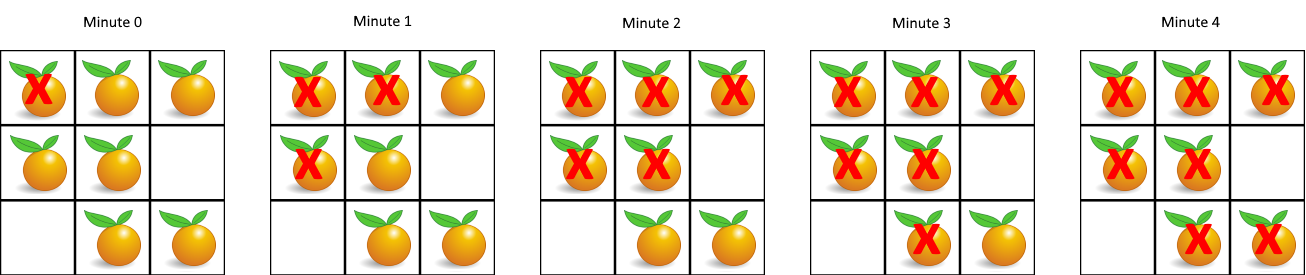``````Input: [[2,1,1],[1,1,0],[0,1,1]]
Output: 4``````

``````class Solution:
def orangesRotting(self, grid: List[List[int]]) -> int:
q = deque()
visited = set()
fresh = 0
time = 0

for i in range(len(grid)):
for j in range(len(grid)):
if grid[i][j] == 2:
q.append((i, j)) # 把所有的腐烂的橘子都当做是起始点
elif grid[i][j] == 1:
fresh += 1

if fresh == 0:
return 0

while q:
for _ in range(len(q)):
i, j = q.popleft()

for x, y in (i + 1, j), (i - 1, j), (i, j + 1), (i, j - 1):
if 0 <= x < len(grid) and 0 <= y < len(grid) and (x, y) not in visited and grid[x][y] == 1:
fresh -= 1
q.append((x, y))
time += 1

return time - 1 if fresh == 0 else -1``````

• 时间复杂度：O(MN)，其中 M 和 N 分别为行数和列数。因为我们最多遍历一次矩阵中的全部元素。
• 空间复杂度：O(MN)，其中 M 和 N 分别为行数和列数。主要为队列的开销。

#### 1091. Shortest Path in Binary Matrix

test cases:

``````Input: [[0,0,0],[1,1,0],[1,1,0]]
Output: 4``````

``````class Solution:
def shortestPathBinaryMatrix(self, grid: List[List[int]]) -> int:
q = deque()
visited = set()
n = len(grid)

if grid == 1 or grid[n - 1][n - 1] == 1:
return -1

q.append((0, 0))
time = 0

while q:
for _ in range(len(q)):
i, j = q.popleft()

if i == n - 1 and j == n - 1:
return time + 1

for x, y in (i + 1, j), (i - 1, j), (i, j + 1), (i, j - 1), (i + 1, j + 1), (i - 1, j - 1), (i + 1, j - 1), (i - 1, j + 1):
if 0 <= x < n and 0 <= y < n and (x, y) not in visited and grid[x][y] == 0:
q.append((x, y))
time += 1

return -1``````

• 时间复杂度：O(MN)，其中 M 和 N 分别为行数和列数。因为我们最多遍历一次矩阵中的全部元素。
• 空间复杂度：O(MN)，其中 M 和 N 分别为行数和列数。主要为队列的开销。

#### 1162. As Far from Land as Possible

test cases:

``````Input: [[1,0,1],[0,0,0],[1,0,1]]
Output: 2``````

``````class Solution:
def maxDistance(self, grid: List[List[int]]) -> int:
q = deque()
visited = set()
n = len(grid)

for i in range(n):
for j in range(n):
if grid[i][j] == 1:
q.append((i, j))

if len(q) == 0 or len(q) == n * n:
return -1

time = 0

while q:
for _ in range(len(q)):
i, j = q.popleft()

for x, y in (i + 1, j), (i - 1, j), (i, j + 1), (i, j - 1):
if 0 <= x < n and 0 <= y < n and (x, y) not in visited and grid[x][y] == 0:
q.append((x, y))
time += 1

return time - 1``````

• 时间复杂度：O(MN)，其中 M 和 N 分别为行数和列数。因为我们最多遍历一次矩阵中的全部元素。
• 空间复杂度：O(MN)，其中 M 和 N 分别为行数和列数。主要为队列的开销。

#### 733. Flood Fill

test cases: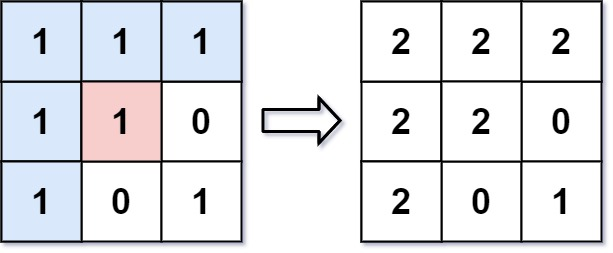``````Input: image = [[1,1,1],[1,1,0],[1,0,1]], sr = 1, sc = 1, color = 2
Output: [[2,2,2],[2,2,0],[2,0,1]]
Explanation: From the center of the image with position (sr, sc) = (1, 1) (i.e., the red pixel), all pixels connected by a path of the same color as the starting pixel (i.e., the blue pixels) are colored with the new color.
Note the bottom corner is not colored 2, because it is not 4-directionally connected to the starting pixel.``````

``````class Solution:
def floodFill(self, image: List[List[int]], sr: int, sc: int, color: int) -> List[List[int]]:
q = collections.deque([(sr, sc)])
old_color = image[sr][sc]
visited = set()
image[sr][sc] = color

while q:
x, y = q.popleft()

for i, j in (x + 1, y), (x - 1, y), (x, y + 1), (x, y - 1):
if 0 <= i < len(image) and 0 <= j < len(image) and image[i][j] == old_color and (i, j) not in visited:
image[i][j] = color
q.append((i, j))
return image``````

• 时间复杂度：O(MN)，其中 M 和 N 分别为行数和列数。因为我们最多遍历一次矩阵中的全部元素。
• 空间复杂度：O(MN)，其中 M 和 N 分别为行数和列数。主要为队列的开销。

#### 490. The Maze

test cases: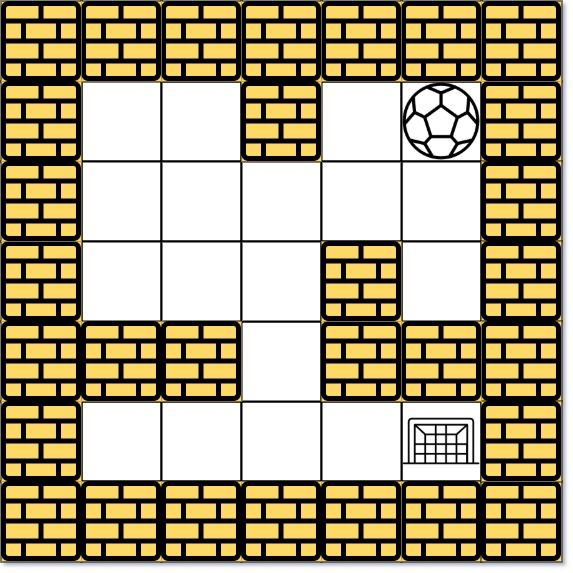``````Input: maze = [[0,0,1,0,0],[0,0,0,0,0],[0,0,0,1,0],[1,1,0,1,1],[0,0,0,0,0]], start = [0,4], destination = [4,4]
Output: true
Explanation: One possible way is : left -> down -> left -> down -> right -> down -> right.``````

``````class Solution:
import collections
def hasPath(self, maze: List[List[int]], start: List[int], destination: List[int]) -> bool:
rows = len(maze)
cols = len(maze)

dir = [1, 0, -1, 0, 1]
visited = [[0 for _ in range(cols)] for _ in range(rows)]
q = deque()
q.append(start)
visited[start][start] = 1

while q:
cur = q.popleft()
if cur == destination and cur == destination:
return True

for i in range(4):
newX = cur
newY = cur

while 0 <= newX < rows and 0 <= newY < cols and maze[newX][newY] != 1:
newX += dir[i]
newY += dir[i + 1]

newX -= dir[i]
newY -= dir[i + 1]

if visited[newX][newY]: continue

q.append([newX, newY])
visited[newX][newY] = 1

return False``````

• 时间复杂度：O(MN)，其中 M 和 N 分别为行数和列数。因为我们最多遍历一次矩阵中的全部元素。
• 空间复杂度：O(MN)，其中 M 和 N 分别为行数和列数。主要为队列的开销。

#### 505. The Maze II

test cases:``````Input: maze = [[0,0,1,0,0],[0,0,0,0,0],[0,0,0,1,0],[1,1,0,1,1],[0,0,0,0,0]], start = [0,4], destination = [4,4]
Output: 12
Explanation: One possible way is : left -> down -> left -> down -> right -> down -> right.
The length of the path is 1 + 1 + 3 + 1 + 2 + 2 + 2 = 12.``````

``````class Solution:
import collections
import sys
def shortestDistance(self, maze: List[List[int]], start: List[int], destination: List[int]) -> int:

rows = len(maze)
cols = len(maze)

dir = [1, 0, -1, 0, 1]
res = [[float('inf') for _ in range(cols)] for _ in range(rows)]
q = deque()
q.append([start, start, 0])

while q:
cur = q.popleft()
if cur >= res[cur][cur]:
continue
res[cur][cur] = cur

for i in range(4):
newX = cur
newY = cur
path = cur
while 0 <= newX < rows and 0 <= newY < cols and maze[newX][newY] == 0:
newX += dir[i]
newY += dir[i + 1]
path += 1
newX -= dir[i]
newY -= dir[i + 1]
path -= 1

q.append([newX, newY, path])

return -1 if res[destination][destination] == float('inf') ``````